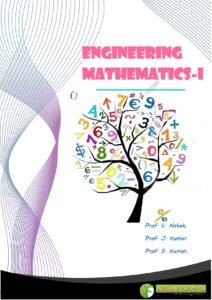You are here
Home > Book > Engineering Mathematics-1 PDF Book

# Book Detail: Engineering Mathematics-I

Engineering mathematics is a branch of applied mathematics concerning mathematical methods and techniques that are typically used in engineering and industry.Language: English

Pages: 368

Author: Prof. C. Nahak, Prof. J. Kumar & Prof. S. Kumar

Price: Free## Outlines of Engineering Mathematics-I

### Module 1: Differential Calculus

Lesson 1: Rolle’s Theorem, Lagrange’s Mean Value Theorem, Cauchy’s Mean Value Theorem

Lesson 2: Taylor’s Theorem / Taylor’s Expansion, Maclaurin’s Expansion

Lesson 3: Indeterminate forms ; L’Hospital’s Rule

Lesson 4: Limit, Continuity of Functions of Two Variables

Lesson 5: Partial and Total Derivatives

Lesson 6: Homogeneous Functions, Euler’s Theorem

Lesson 7: Composite and Implicit functions for Two Variables

Lesson 8: Derivative of Higher Order

Lesson 9: Taylor’s Expansion for Function of Two Variables

Lesson 10: Maximum and Minimum of Function of Two Variables

Lesson 11: Lagrange’s Multiplier Rule / Constrained Optimization

Lesson 12: Convexity, Concavity and Points of Inflexion

Lesson 13: Curvature

Lesson 14: Asymptotes

Lesson 15: Tracing of Curves

### Module 2: Integral Calculus

Lesson16: Improper Integral

Lesson17. Test for Convergence

Lesson 18: Rectification

Lesson 19: Volume and Surface of Revolution

Lesson 20: Double Integration

Lesson 21: Triple Integration

Lesson 22: Area & Volume using Double and Triple Integration

Lesson 23: Gamma Function

Lesson 24: The Beta Function

### Module 3: Ordinary Differential Equations

Lesson 25: Introduction

Lesson 26: Differential Equations of First Order

Lesson 27: Linear Differential Equation of First Order

Lesson 28: Exact Differential Equations of First Order

Lesson 29: Exact Differential Equations : Integrating Factors

Lesson 30: Linear Differential Equations of Higher Order

Lesson 31: Linear Differential Equations of Higher Order

Lesson 32: Linear Differential Equations of Higher Order (Cont.)

Lesson 33: Method of Undetermined Coefficients

Lesson 34: Method of Variation of Parameters

Lesson 35: Equations Reducible to Linear Differential Equations with Constant Coefficient

Lesson 36: Methods for Solving Simultaneoous Ordinary Differential Equations

Lesson 37: Series Solutions about an Ordinary Point

Lesson 38: Series Solutions about an Ordinary Points (Cont.)

Lesson 39: Series Solutions about a Regular Singular Point

Lesson 40: Series Solutions about a Regular Singular Point (Cont..)

### Module 4: Vector Calculus

Lesson 41: Introduction

Lesson 42: Gradient and Directional Derivatives

Lesson 43: Divergence and Curl

Lesson 44: Line Integral

Lesson 45: Green’s Theorem in the Plane

Lesson 46: Surface Integral

Lesson 47: Stokes’s Theorem

Lesson 48: Divergence Theorem of Gauss

For Daily Update follow us at:

Disclaimer: The information on this website does not warrant or assume any legal liability or responsibility for the accuracy, completeness or usefulness of the courseware contents.

The contents are provided free for noncommercial purpose such as teaching, training, research, extension and self learning.

1.Jairous mulenga says: